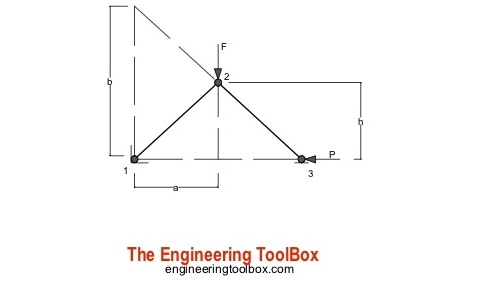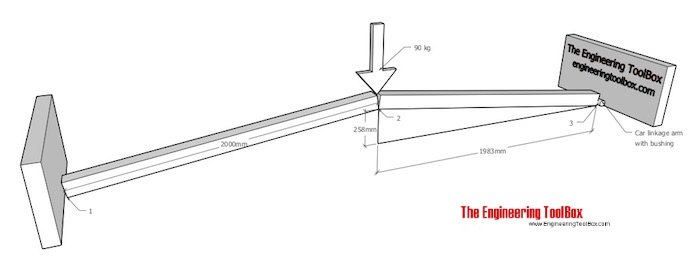Engineering ToolBox - Resources, Tools and Basic Information for Engineering and Design of Technical Applications!

# Toggle Joint

## A toggle joint mechanism can be used to multiply force.The toggle mechanism is made of two arms linked together. Point 1 is fixed, point 3 can move horizontally (as indicated in this principal drawing). The effort force acts vertically in point 2.

The horizontal force acting in point 3 can be calculated for arms with equal or unequal lengths.

### Arms with equal lengths

P = F a / 2 h                            (1)

where

P = horizontal force in point 3 (N, lb)

F = effort force (N, lb)

a = length (m, ft)

h = height (m, ft)

### Arms with unequal lengths

P = F a / b                             (2)

where

b = length (m, ft)

### Example - An Improvised Press for Replacing Car Bushings

A press to remove/refit bushings in car linkage arms can be improvised with two pieces of lumber:The effort force acting on the toggle joint (in point 2) with a person with weight 90 kg can be calculated as

F = (90 kg) (9.81 m/s2)

= 883 N

The force acting on the bush (in point 3) can be calculated as

P = F a / 2 h

= (883 N) (1983 mm) / (2 (258 mm))

= 3393

The force ratio is

Fr = (3393 N) / (883 N)

= 3.8

- the initial effort force is amplified near four times

### Toggle Joint Calculator

This calculator can be used to calculate the resulting force in a toggle joint with arms with equal lengths. The calculator can be used for imperial and metric units as long as the use of units are consistent.

Note that kg is the SI unit of mass - check the difference between mass and weight (force).

## Related Topics

• ### Mechanics

Forces, acceleration, displacement, vectors, motion, momentum, energy of objects and more.

## Related Documents

• ### Acceleration of Gravity and Newton's Second Law

Acceleration of gravity and Newton's Second Law - SI and Imperial units.
• ### Car - Required Power and Torque

Power, torque, efficiency and wheel force acting on a car.
• ### Car - Traction Force

Adhesion and tractive force between car wheel and surface.
• ### Car Acceleration

Car acceleration calculator.
• ### Force

Newton's third law - force vs. mass and acceleration.
• ### Force Ratio

The force ratio is the load force versus the effort force.
• ### Gear Reducing Formulas

Output torque, speed and horsepower with gears.
• ### Gears

Gears effort force vs. load force.
• ### Levers

Use levers to magnify forces.
• ### Movement Ratio

Machines and movement ratio (velocity ratio).
• ### Pulleys

Pulleys, blocks and tackles.
• ### Screw Jack - Effort Force vs. Load

Screw jacks and effort forces.

## Engineering ToolBox - SketchUp Extension - Online 3D modeling!

Add standard and customized parametric components - like flange beams, lumbers, piping, stairs and more - to your Sketchup model with the Engineering ToolBox - SketchUp Extension - enabled for use with older versions of the amazing SketchUp Make and the newer "up to date" SketchUp Pro . Add the Engineering ToolBox extension to your SketchUp Make/Pro from the Extension Warehouse !

We don't collect information from our users. More about

## Citation

• The Engineering ToolBox (2018). Toggle Joint. [online] Available at: https://www.engineeringtoolbox.com/toggle-joint-d_2077.html [Accessed Day Month Year].

Modify the access date according your visit.

9.29.12